Case Based Questions (MCQ)

Chapter 8 Class 10 Introduction to Trignometry
Serial order wise

This question is inspired from  Question 24 CBSE Class 10 Sample Paper for 2020 Boards

## 'Skysails' is that genre of engineering science that  uses extensive utilization of wind energy to move  a vessel in the sea water. The sky sails technology  allows the towing kite to gain a height of anything  between 100 m to 300 m. The sailing kite is made  in such a way that it can be raised to its proper  elevation and then brought back with the help of  a telescopic mast that enables the kite to be raised  properly and effectively.  Based on the following figure related to sky sailing  answer the questions: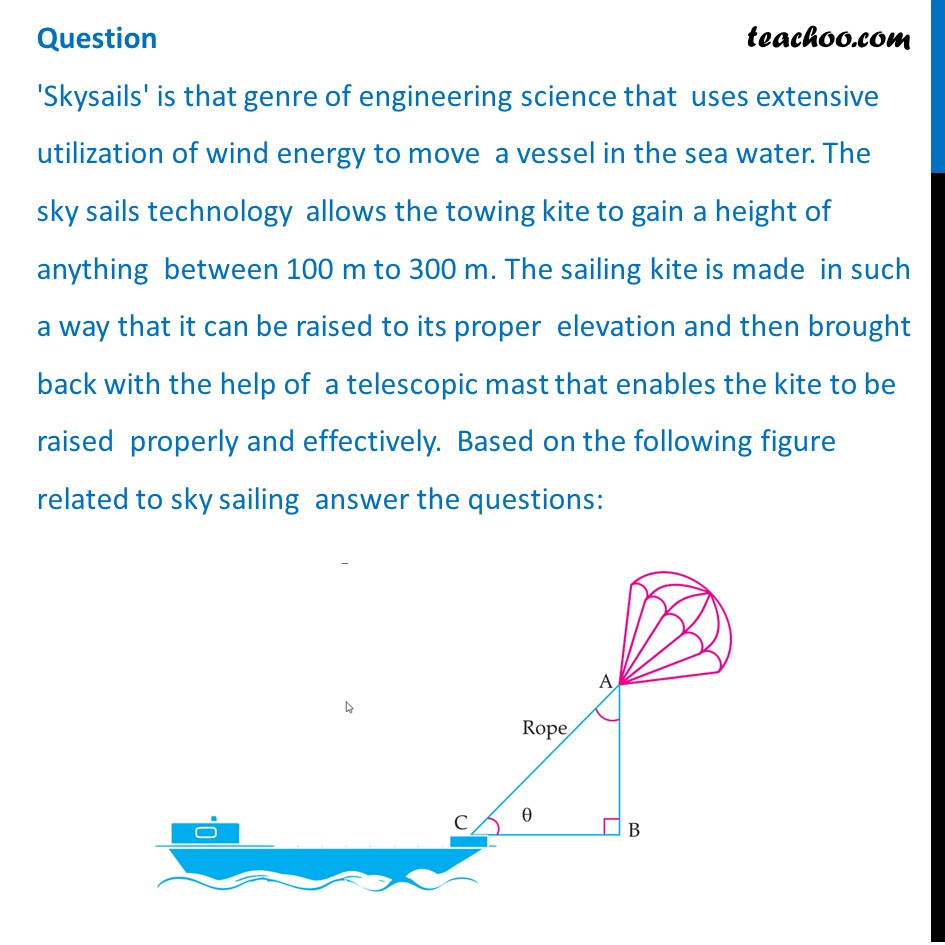## (c) 60°                             (d) None of these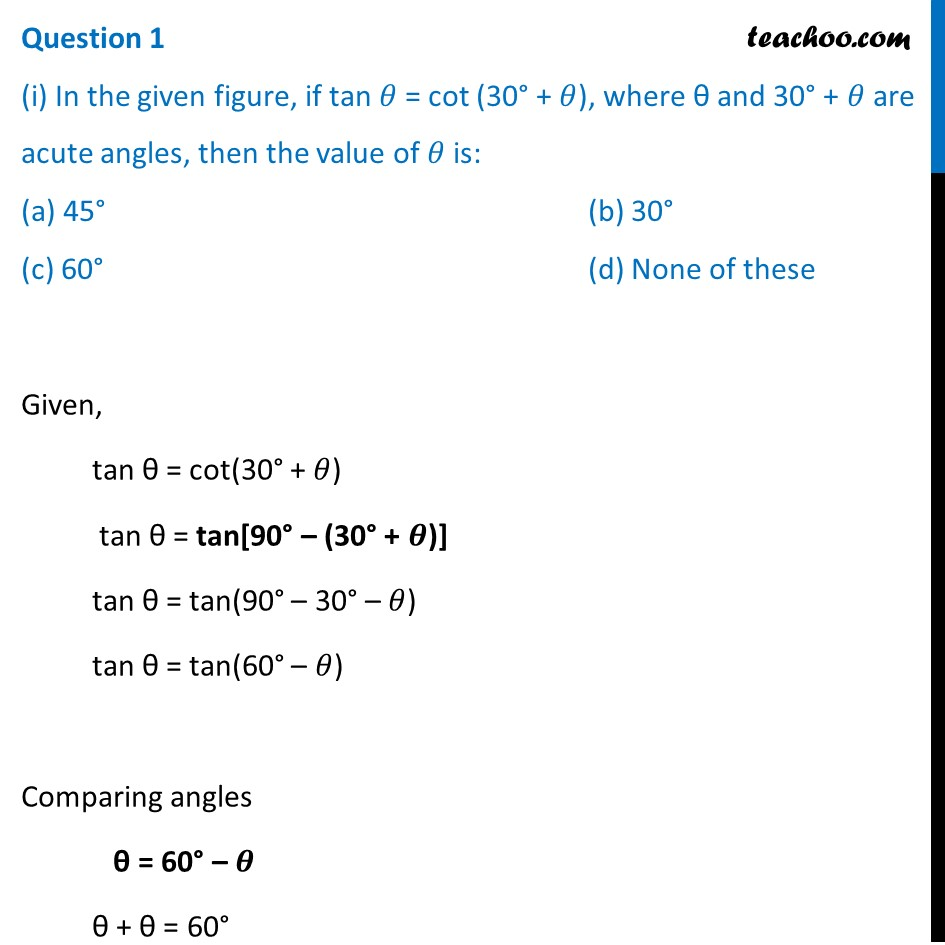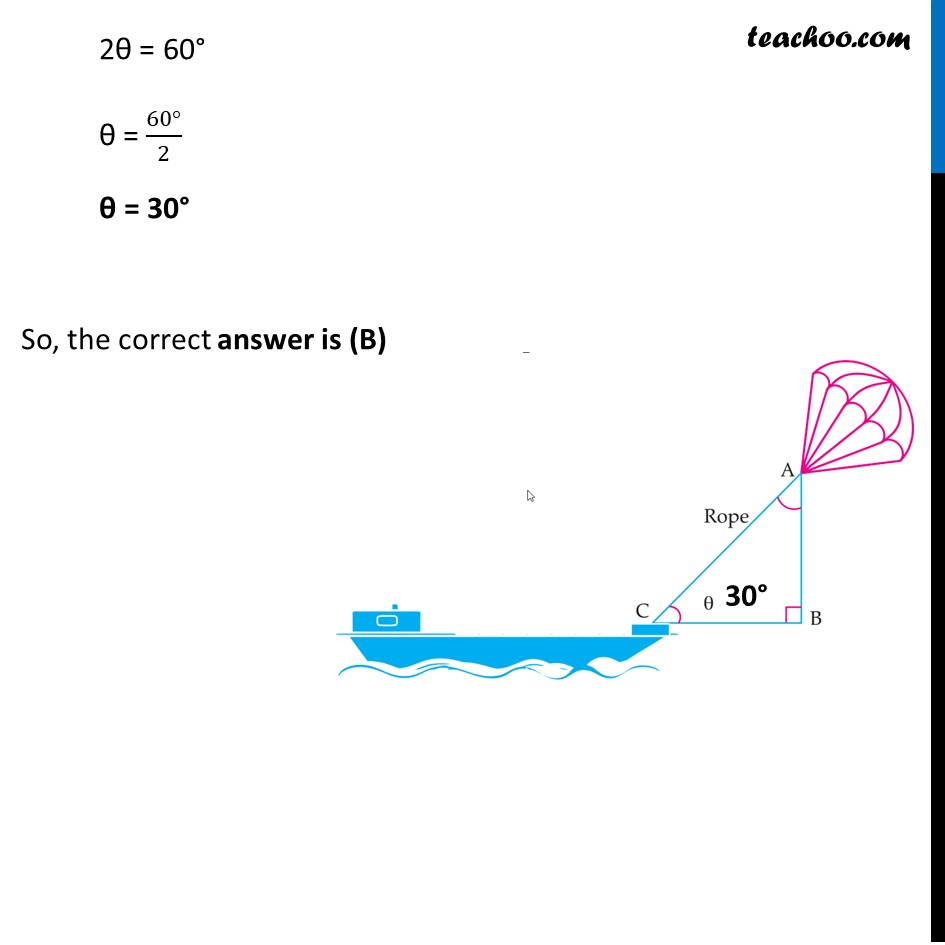## (C)  1                         (D) 1/3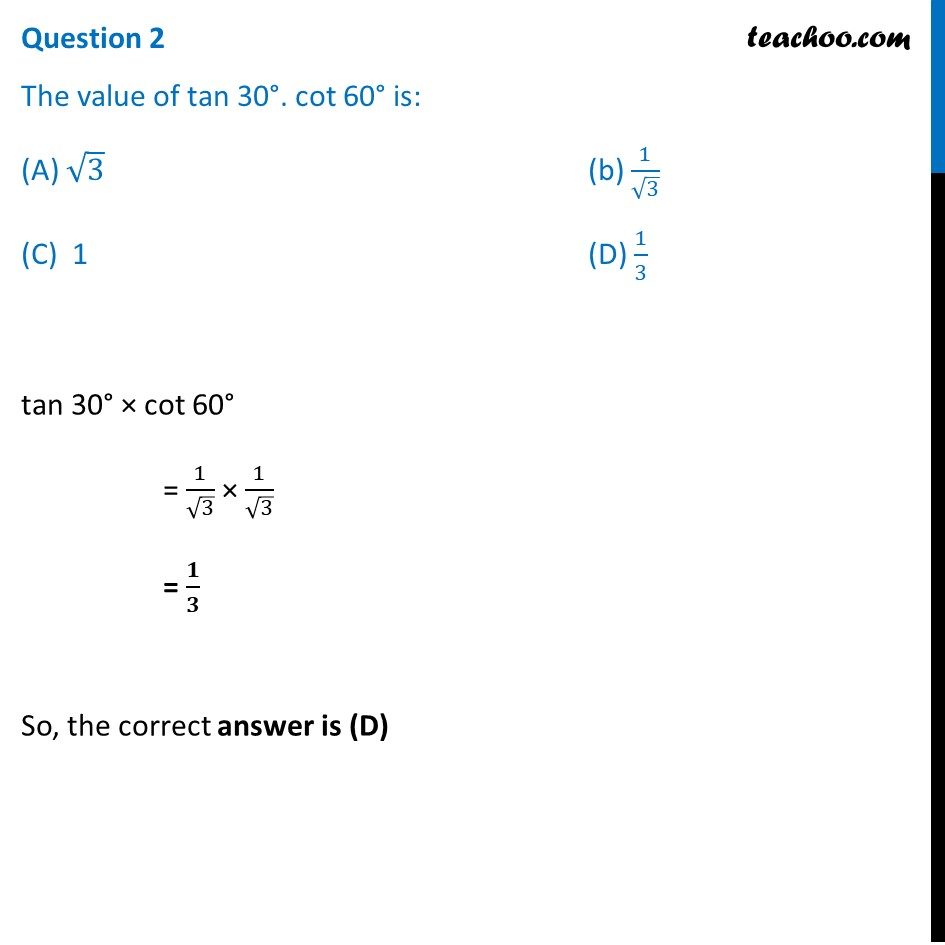## (c) 100 m                                 (d) 200 m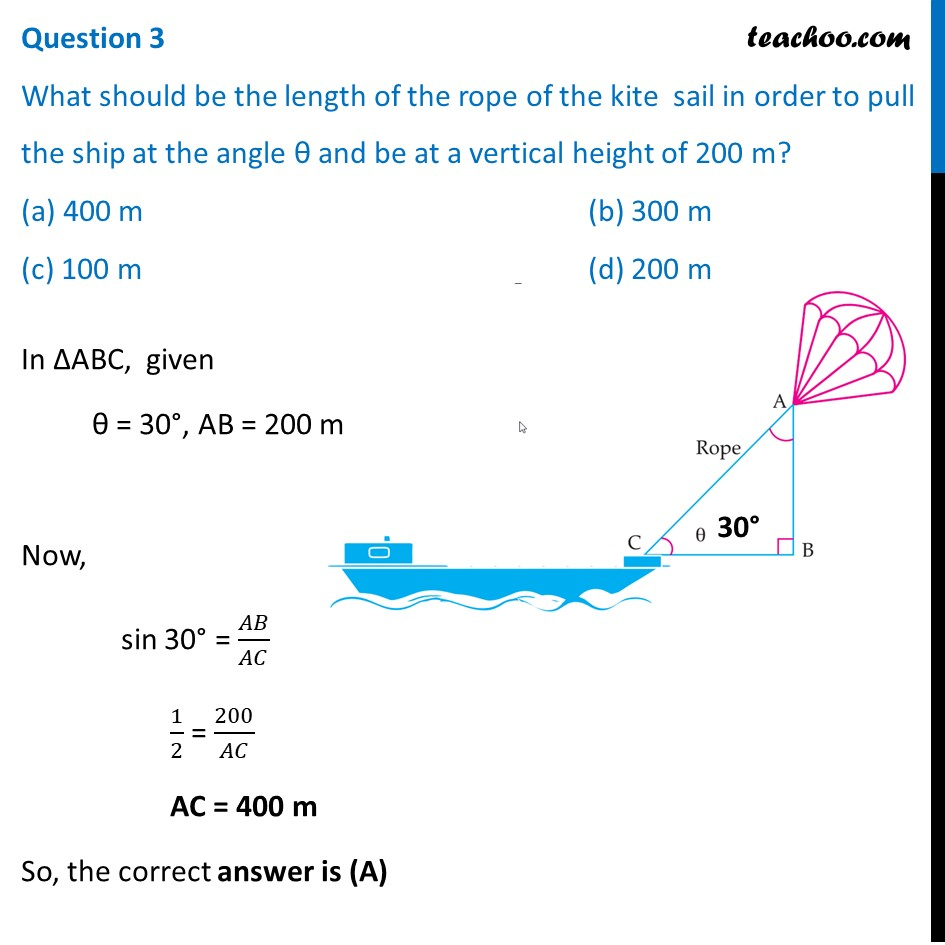## (C) 2                                     (D) 4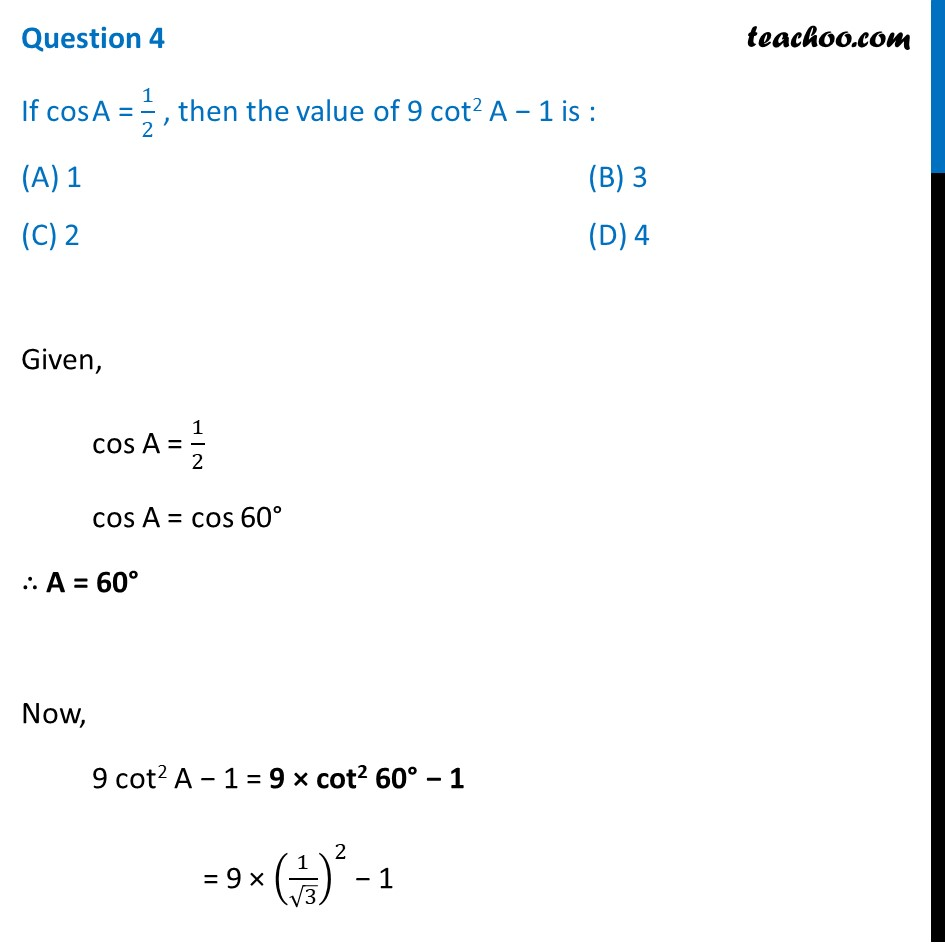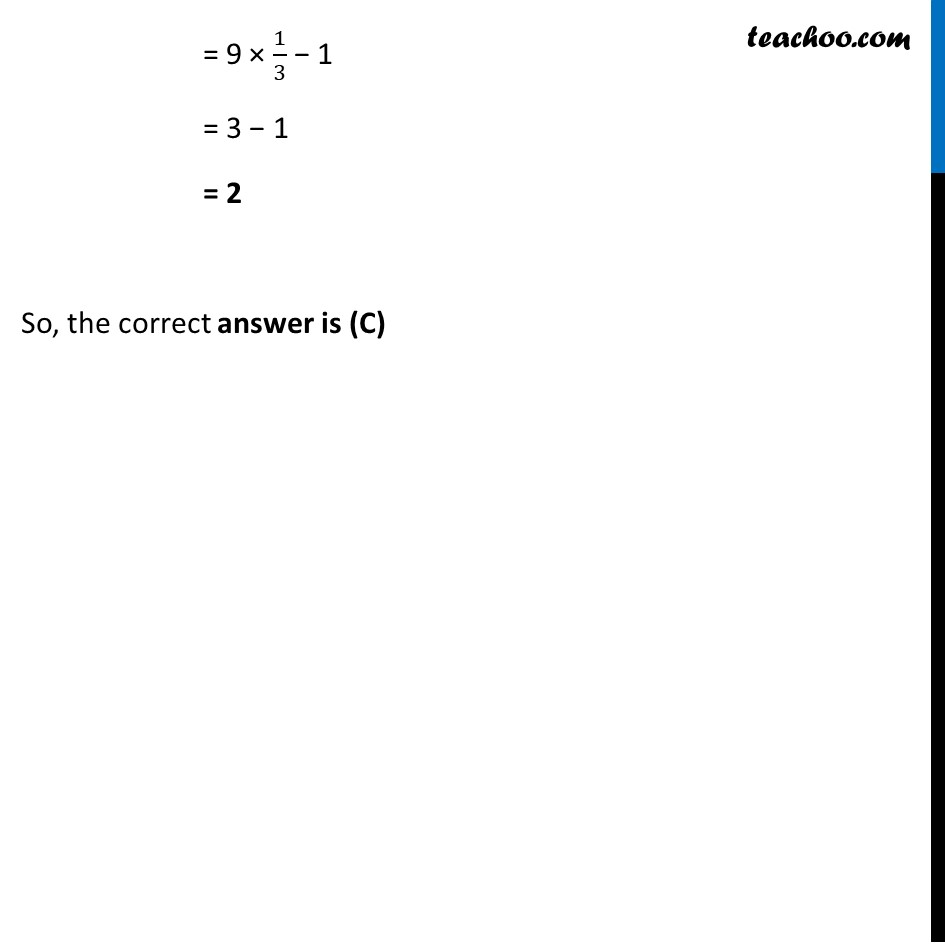## (c) 3                                                     (d) 4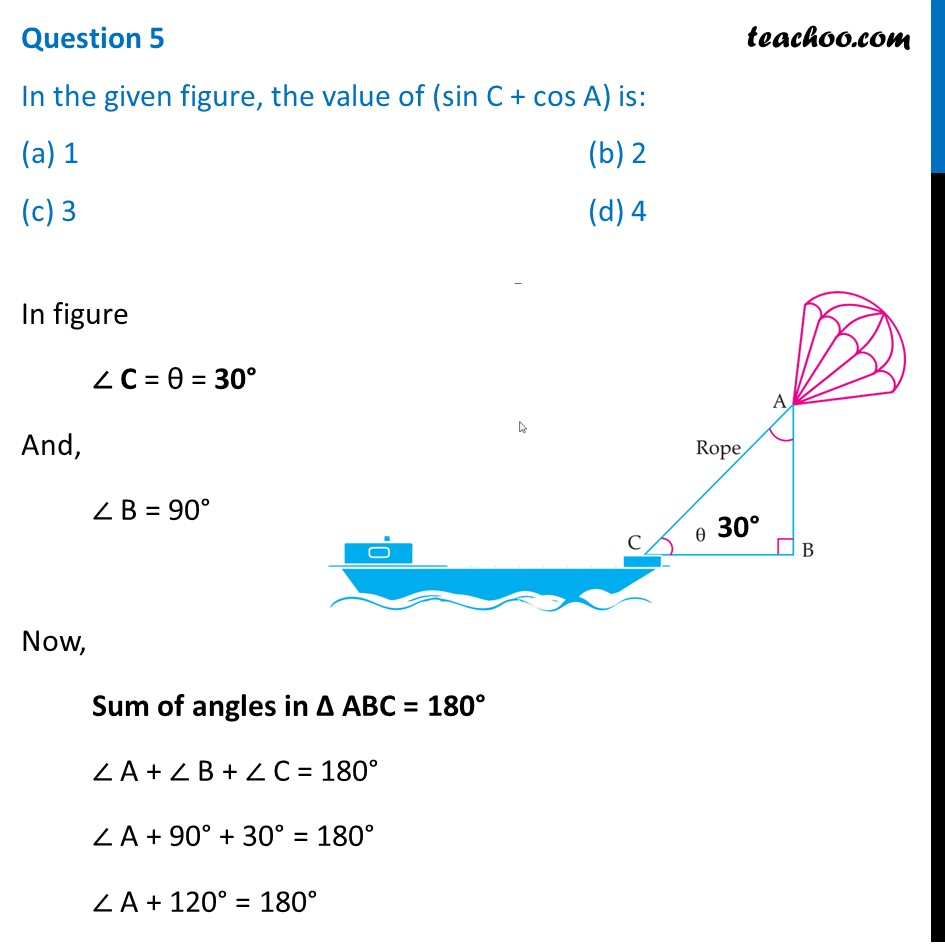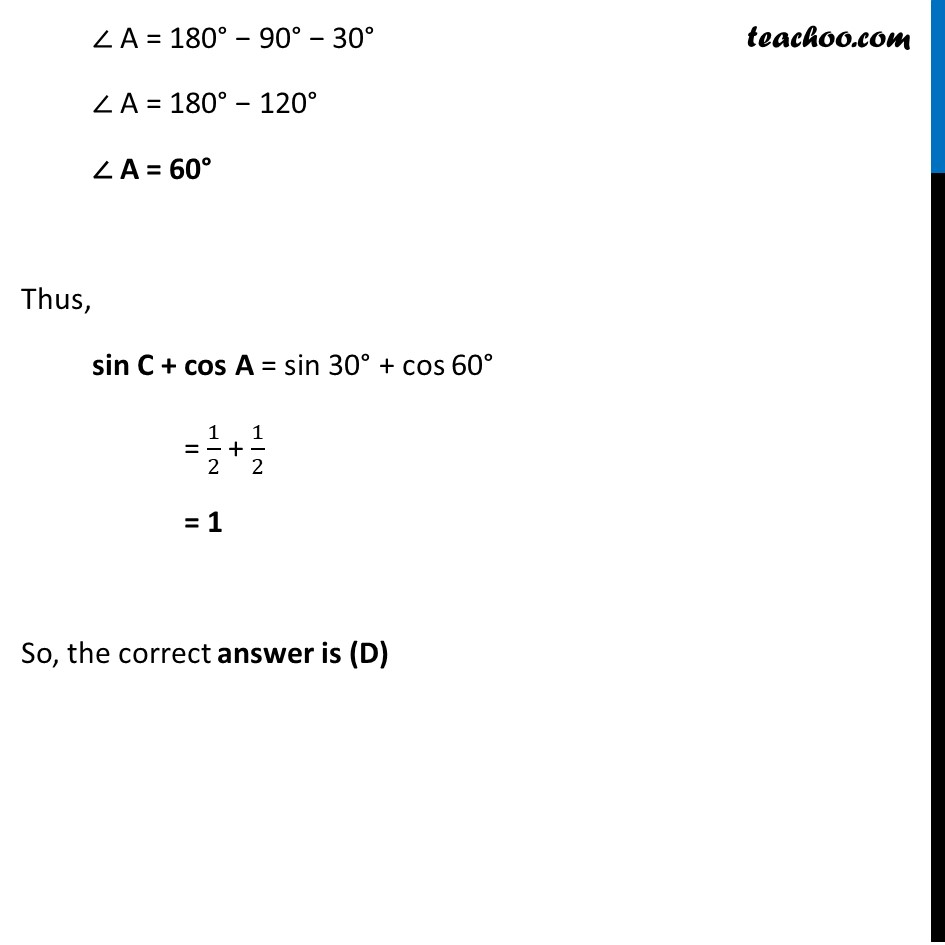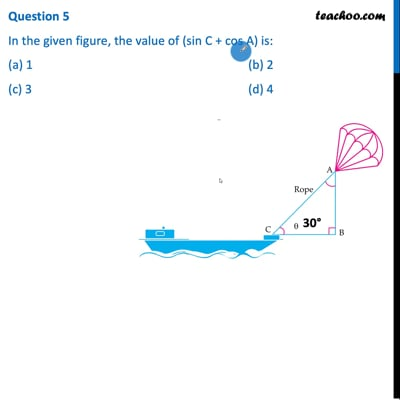This video is only available for Teachoo black users

Maths Crash Course - Live lectures + all videos + Real time Doubt solving!

### Transcript

Question 'Skysails' is that genre of engineering science that uses extensive utilization of wind energy to move a vessel in the sea water. The sky sails technology allows the towing kite to gain a height of anything between 100 m to 300 m. The sailing kite is made in such a way that it can be raised to its proper elevation and then brought back with the help of a telescopic mast that enables the kite to be raised properly and effectively. Based on the following figure related to sky sailing answer the questions: Question 1 (i) In the given figure, if tan 𝜃 = cot (30° + 𝜃), where θ and 30° + 𝜃 are acute angles, then the value of 𝜃 is: (a) 45° (b) 30° (c) 60° (d) None of these Given, tan θ = cot(30° + 𝜃) tan θ = tan[90° – (30° + 𝜽)] tan θ = tan(90° – 30° – 𝜃) tan θ = tan(60° – 𝜃) Comparing angles θ = 60° – 𝜽 θ + θ = 60° 2θ = 60° θ = (60°)/2 θ = 30° So, the correct answer is (B) 2θ = 60° θ = (60°)/2 θ = 30° So, the correct answer is (B) 2θ = 60° θ = (60°)/2 θ = 30° So, the correct answer is (B) Question 2 The value of tan 30°. cot 60° is: (A) √3 (b) 1/√3 (C) 1 (D) 1/3 tan 30° × cot 60° = 1/√3 × 1/√3 = 𝟏/𝟑 So, the correct answer is (D) Question 3 What should be the length of the rope of the kite sail in order to pull the ship at the angle θ and be at a vertical height of 200 m? (a) 400 m (b) 300 m (c) 100 m (d) 200 m In ∆ABC, given θ = 30°, AB = 200 m Now, sin 30° = 𝐴𝐵/𝐴𝐶 1/2 = 200/𝐴𝐶 AC = 400 m So, the correct answer is (A) Question 4 If cos A = 1/2 , then the value of 9 cot2 A − 1 is : (A) 1 (B) 3 (C) 2 (D) 4 Given, cos A = 1/2 cos A = cos 60° ∴ A = 60° Now, 9 cot2 A − 1 = 9 × cot2 60° − 1 = 9 × (1/√3)^2 − 1 Given, cos A = 1/2 cos A = cos 60° ∴ A = 60° Now, 9 cot2 A − 1 = 9 × cot2 60° − 1 = 9 × (1/√3)^2 − 1 = 9 × 1/3 − 1 = 3 − 1 = 2 So, the correct answer is (C) Question 5 In the given figure, the value of (sin C + cos A) is: (a) 1 (b) 2 (c) 3 (d) 4 In figure ∠ C = θ = 30° And, ∠ B = 90° Now, Sum of angles in Δ ABC = 180° ∠ A + ∠ B + ∠ C = 180° ∠ A + 90° + 30° = 180° ∠ A + 120° = 180° ∠ A = 180° − 90° − 30° ∠ A = 180° − 120° ∠ A = 60° Thus, sin C + cos A = sin 30° + cos 60° = 1/2 + 1/2 = 1 So, the correct answer is (D)